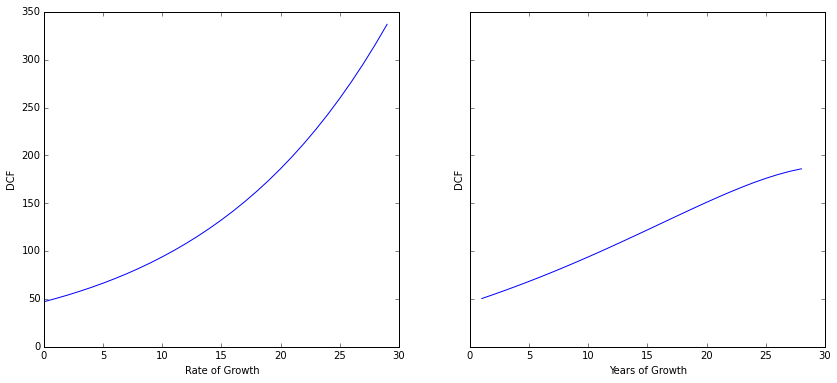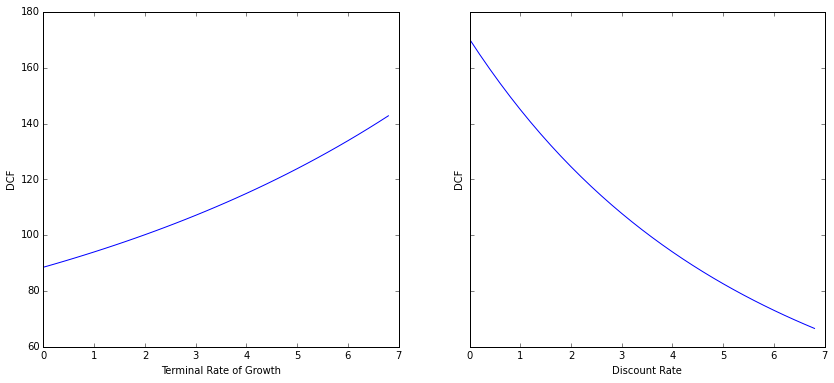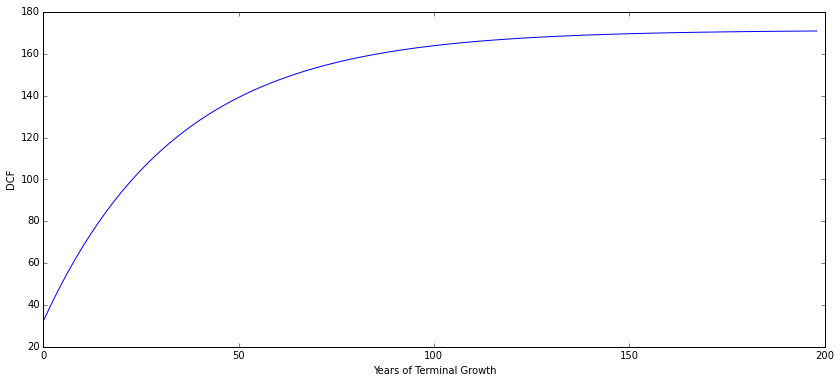# An Introduction to Discounted Cash Flow: Visa Inc

Last Updated: Feb 2016

Discounted Cash Flow (DCF) analysis is one of the most standard ways to value a company. A DCF involves 3 steps:

• Predict cash flows for the next few years (easier said than done).
• Identify an appropriate discount rate for future earnings (since future earnings are less certain than current earnings).
• Sum the series of discounted future cash flows.

The first step can be made arbitrarily complex by considering multitudes of different factors. In this document, we stick to a simple cash flow growth model.

## An example: Moo's Ice Cream

Let's run through an example with a fictional ice-cream company - Moo's ice cream. Let us assume that Moo's, like other good businesses, returns cash to the owners each year. The cash that an owner can safely pull out of any business is termed "Free Cash Flow", and is simply operating profits (sales minus cost of ice cream, salaries, rent and other operating expenditures) net of any capital expenditures needed to sustain the business (replacement and repair of freezers etc..). DCF theory tells us that the value of Moo's is simply the sum of discounted future cash flows.

Let us make some assumptions.

Suppose that my intimate knowledge of Moo's allows me to project the expected free cash flows for the next decade (let us not worry how just yet):

Year Expected Cash Flow
1 2.5 million dollars
2 2.7 million dollars
3 2.7 million dollars
4 3.0 million dollars
5 3.1 million dollars
6 3.3 million dollars
7 3.7 million dollars
8 3.9 million dollars
9 4.5 million dollars
10 5.0 million dollars

Further, let us assume that I do not expect any growth after the next decade and that I expect Moo's to survive for only 20 years (the ice cream business has become competitive!).

Assuming Moo's has 1 million shares outstanding (and assuming that this is unlikely to change), we may write the cashflows per share as:

In :
cashflows = [2.5, 2.7, 2.7, 3.0, 3.1, 3.3, 3.7, 3.9, 4.5, 5.0] + [5.0] * 10


Any decision to invest money must consider a notion of an opportunity cost of investing in a risk-free manner. This is incorporated as the discount rate in the DCF analysis. For this example, let us consider the opportunity cost to be 4% per year (more on this later).

Then, the sum of discounted cash flows for Moo's will be:

$\frac{2.5}{1.04^0} + \frac{2.7}{1.04^1} + \frac{2.8}{1.04^2} + \ldots + \frac{5.0}{1.04^9} + \frac{5.0}{1.04^{10}} + \ldots + \frac{5.0}{1.04^{19}}$

or in other words:

$DCF = \sum_{i=0}^{19} \frac{cashflows[i]}{1.04^i}$

We may compute this as follows:

In :
dcf = 0
for i in range(0, len(cashflows)):
dcf += cashflows[i] / (1.04)**i

round(dcf, 2)

Out:
56.8


This gives us a fair value of $56 per share. Obviously, given the many assumptions I have made, I would like to buy shares of Moo's with a 30% margin of safety, for a price of$40.

This is all there is to the mathematics of DCF. As with many things in the real world, it is simple but not easy (for reasons that we will see below).

## Evaluating Visa Inc

### The Unknowns

There are 3 main variables in any DCF analysis:

• How many years can I reasonably expect this business to exist?
• What are the future cash flows?
• What is an appropriate discount rate?

We shall take each into consideration and go about making reasonable estimates for Visa Inc.

### How many years can I reasonably expect a business to exist?

This question is qualitative in nature, and addressing this requires a lot of reading, familiarity with the industry, and armchair thought.

At the heart of this question lies the idea of a competitive advantage or "Economic Moat". An Economic Moat refers to the ability of a business to maintain competitive advantages over it's competitors in the long term. Moats can take many forms including:

• Economies of scale - Walmart
• High Switching costs - Oracle / IBM
• Expensive to build an alternative - Amazon
• Regulatory advantages - Banks like JP Morgan
• Brand power - Coca Cola / Kleenex
• Network Effects - Facebook

Two good sources of reading about Moats are The little book that builds wealth by Pat Dorsey and Competition Demystified by Bruce Greewald. Phil Fisher of Common Stocks and Uncommon Profits is also a very good reading for learning about key factors that influence growth in businesses. If you're feeling more adventurous, Competitive Strategy by Michael Porter is the bible.

#### Estimating the lifetime of Visa Inc

Visa Inc is a stable, growing business, with an easy to understand business model. Visa acts as a payment gateway between the customer's bank and the merchant's bank and charges a fraction of all money transacted. The world currently has around ~600M credit cards with plenty of room for growth. Regulatory problems around the world prevent new entrants from cropping up. It is not inconceivable to imagine a business like Visa to last for at least 2-3 more decades.

No business is without risks. In particular, given the ubiquity of credit cards, and the oligopoly forming in payment providers, organizations like the European Union are trying to cap and actively reduce the surcharge that these organizations can take.

### Estimation of Future Cash Flows

This is perhaps the hardest part of Discounted Cash Flow analysis. This is where the story dictates the final result. Small changes to assumptions in growth of user base or reduction of capital expenditures yield widely varying results after 10 years of simulation.

We will circumvent this complexity by using a cash flow growth model. In this model, we project the cash flows to grow at a constant rate during the growth period after which we assume terminal (slow or zero) growth for the remaining life of the business. This may sound too simple to work. However, in Valuation, it is advantageous to to use a simpler model over a complex one:

• As with all engineering, it is important to be directionally right than precisely wrong. Keeping estimations simple allows us to play with a range of scenarios.
• The more time we spend analysing something, the more we likely we are to feel an illusion of certainity. By intentionally sticking to an absurdly simple valuation procedure, we explicitly acknowledge the many unknowns, and focus instead on the assumptions in our analysis.
• Using a simple cash flow growth model only makes sense when we can reasonably predict a rate of growth. This forces us to focus on stable predictable businesses within our circle of competencies.

Currently, (as of Feb 2016), Visa earns $2.49 per share as free cash flow (for all data, I use morningstar's 10 year key ratios). By sticking to this simple model, we can spend time thinking about Visa's likely growth rate. Historically, Visa's net income has grown at ~30%. More recently, as Visa has become a larger company, the growth has slowed down to ~15%. Looking at market share, we can see that Visa currently captures ~70% of the debit card network, and ~45% of the credit card network. There are only ~600 million cards in circulation, a number we can expect to increase multifold in the future. As another tailwind to Visa's business, mobile payment platforms like Apple Pay also use Visa as a payment gateway, unlocking another source of growth. It is not unreasonable to expect a 10% year on year growth for Visa over the next 10 years. A 10% growth means that visa's profits double every seven years or so, leaving us with a ~3x increase in profits / cash flow by 2026. Let us go with this assumption for now and revisit other growth rates later on. Finally, we shall assume that Visa stops growing altogether (or grows very slowly at 1%) for the next 2 decades. This gives us cash flows of: In : growth_cashflows = [2.49 * (1.10)**i for i in range(10)] # Cashflows during growth stage terminal_cashflows = [growth_cashflows[-1] * (1.01)**(i+1) for i in range(20)] # Cashflows during terminal growth cashflows = growth_cashflows + terminal_cashflows # Pretty print cashflows [("Year %d"%(i+1), round(x, 2)) for (x, i) in zip(cashflows, range(len(cashflows)))]  Out: [('Year 1', 2.49), ('Year 2', 2.74), ('Year 3', 3.01), ('Year 4', 3.31), ('Year 5', 3.65), ('Year 6', 4.01), ('Year 7', 4.41), ('Year 8', 4.85), ('Year 9', 5.34), ('Year 10', 5.87), ('Year 11', 5.93), ('Year 12', 5.99), ('Year 13', 6.05), ('Year 14', 6.11), ('Year 15', 6.17), ('Year 16', 6.23), ('Year 17', 6.29), ('Year 18', 6.36), ('Year 19', 6.42), ('Year 20', 6.49), ('Year 21', 6.55), ('Year 22', 6.62), ('Year 23', 6.68), ('Year 24', 6.75), ('Year 25', 6.82), ('Year 26', 6.88), ('Year 27', 6.95), ('Year 28', 7.02), ('Year 29', 7.09), ('Year 30', 7.16)]  ### Estimation of Discount Rates Literature suggests using the current risk free rate (10 Year US Treasury Government). Given how low the risk free rate is currently, using the 10 year AAA corporate bond rate can be a better idea. Yahoo Finance has a list of corporate bond rates. As of the time of writing, the 10Yr treasury yield is just over 2%, and the 10 Yr AAA corporate bond rate is around 2.7%. For me, however, as an individual investor, my decision to invest (or not) is based on what the broader market may otherwise offer me, i.e, a hurdle rate or opportunity cost. Given S&P 500 P/Es around 25 and dividend yield around 2%, a 10 year return of 4% does not seem unreasonable in the S&P 500. For this reason, let will use a discount rate of 4%. This also gives me a more conservative intrinsic value (upon which I must still apply a margin of safety). Do note that this can get complicated when valuing companies with cash flows from currencies across the world. Different currencies have different risk free rates, and if one is not careful, it is very easy to compute cash flows in one regime, but discount for risk in another. As always, the goto source is Ashwath Damodaran. ### Calculation of Intrinsic Value With all the assumptions made, the calculation of the the intrinsic value is fairly simple: In : dcf = sum([cashflows[i] / (1.04)**i for i in range(len(cashflows))]) round(dcf, 2)  Out: 94.01  Our assumptions give us an intrinsic value of ~$94 . Given our many assumptions, we must insist on a healthy margin of safety (~30%). This gives us a price of ~$65 when VISA becomes attractive. ## Sensitivity to Inputs It is very important to understand how sensitive growing businesses are to the assumptions of growth, and lifetime of estimation. The easiest way to understand it is to plot how the final DCF varies with changes in any of the inputs. To get started, let us put all of our computation in a function: In : def VisaDCF(growth_rate, growth_years, terminal_rate, terminal_years, discount_rate): growth_cashflows = [2.49 * (1 + growth_rate / 100.0)**i for i in range(growth_years)] terminal_cashflows = [growth_cashflows[-1] * (1+terminal_rate /100.0)**(i+1) for i in range(terminal_years)] cashflows = growth_cashflows + terminal_cashflows return sum([cashflows[i] / (1 + discount_rate / 100.0)**i for i in range(len(cashflows))])  To verify the function, we shall revaluate our current assumptions: In : round(VisaDCF(10, 10, 1, 20, 4), 2)  Out: 94.01  Let us begin by plotting DCF versus each of the following: * Growth Rate * Number of years (out of 30) that VISA grows for * Terminal Growth Rate * Discount Rate In : %matplotlib inline import matplotlib import numpy as np import matplotlib.pyplot as plt def Plot(ax, f, range_list, xlabel, ylabel): values = [f(x) for x in range_list] ax.plot(range_list, values) ax.set_xlabel(xlabel) ax.set_ylabel(ylabel) return f, (ax1, ax2) = plt.subplots(1, 2, sharey=True, figsize=(14, 6)) Plot(ax1, lambda g: VisaDCF(g, 10, 1, 20, 4), range(0, 30), 'Rate of Growth', 'DCF') Plot(ax2, lambda n: VisaDCF(10, n, 1, 30-n, 4), range(1, 29), 'Years of Growth', 'DCF') f, (ax1, ax2) = plt.subplots(1, 2, sharey=True, figsize=(14, 6)) Plot(ax1, lambda t: VisaDCF(10, 10, t, 20, 4), np.arange(0, 7, 0.2), 'Terminal Rate of Growth', 'DCF') Plot(ax2, lambda d: VisaDCF(10, 10, 1, 20, d), np.arange(0, 7, 0.2), 'Discount Rate', 'DCF') plt.show()DCF is very sensitive to the rate of growth, terminal rate of growth, and discount rate. As you can see even choosing a 2% discount rate over a 4% increases the DCF from$90 to $120. To avoid perturbations, I enforce the following rules on myself: • Set terminal rate of growth to < 2 %. • Never allow for more than 10 years of growth (who knows what can go wrong?). • Use the same discount rate consistently across various valuations. In particular, using an implied discount rate from the current Shiller PE is one way to look for outperformance of a stock (compared to the index). While these do not elimnate the possibility of baking in overly exuberant assumptions, they do serve to lessen inflation of intrinsic value from non obvious sources. ### An important implicit factor: Lifetime of terminal growth I would like to highlight a key factor that is often negelcted when talking about the intrinsic values, the lifetime of terminal growth. It is perhaps the most hideous source of overly optimistic forecasts as many theoretical treatments of DCF simply consider an infinite horizon and this assumption is implicit. However, we know, in real life, that no business lasts forever. This matters a lot because the sum of infinite cash flows and those truncated a decade or two differ significantly: In : f, ax1 = plt.subplots(1, 1, sharey=True, figsize=(14, 6)) Plot(ax1, lambda t: VisaDCF(10, 10, 1, t, 4), np.arange(0, 200, 2), 'Years of Terminal Growth', 'DCF') plt.show()Our estimate of$94 is a mere 55% of the intrinsic value obtained by assuming an infinite horizon (~$170). The difference is significant, but also crops up from a factor that is not often talked about. In fact, this is precisely why moats are so important. In my analysis, I always assume only a decade of terminal growth. It takes an exceptional business like Visa breaks this rule, (and even then, I have conversatively assumed only two decades). ## Implied Growth Rate Apart from using DCF to find intrinsic value itself, we may also use DCF to find the growth rate implied by the current price. i.e,, what growth rate must be used in the DCF analysis to obtain the current price. This procedure can be very useful in practice as it is often easier to reason about growth rates than prices. Visa is a good example. Consider the current market price of ~$71. Let us proceed to find out what growth rate must be input to the DCF model to imply the current price as a fair value. We can perform a binary search since the DCF vs Rate of Growth curve is monotonic. For simplicity, we are going to guess different growth rates. The above curve shows that the implied growth is in the range [5.5%, 7%]:

In :
for g in np.arange(5.5, 7.0, 0.2):
print g,"% -> DCF: ", round(VisaDCF(g, 10, 1, 20, 4), 2)

5.5 % -> DCF:  68.84
5.7 % -> DCF:  69.8
5.9 % -> DCF:  70.77
6.1 % -> DCF:  71.76
6.3 % -> DCF:  72.76
6.5 % -> DCF:  73.77
6.7 % -> DCF:  74.8
6.9 % -> DCF:  75.85



We can see that the market is currently implying a growth rate of 6% as per our assumptions. Accounting for a margin of safety, the market is implying that Visa will grow at 8-9% over the next 10 years. In Visa's case, it is probably easier to answer this implied growth question: "Do we expect Visa to grow at >8-9% over the next 10 years?".

I personally think so!